Function Graph

Parent topic:
Functions
•Remote Learning Templates for GeoGebra Classroom

Activity

GeoGebra Classroom Activities

•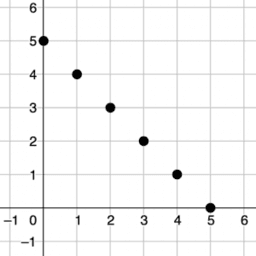Introducing Graphs of Proportional Relationships: IM 7.2.10

Book

GeoGebra Classroom Activities

•Graph the Line

Activity

Steve Phelps

•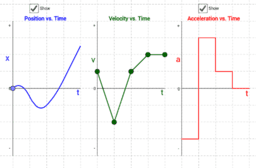Position, Velocity, and Acceleration vs. Time Graphs

Activity

Tom Walsh

•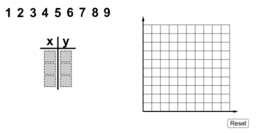Make a Linear Function

Activity

John Ulbright

•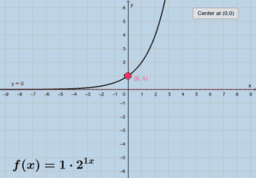Exponential Functions: Graphs

Activity

Tim Brzezinski

•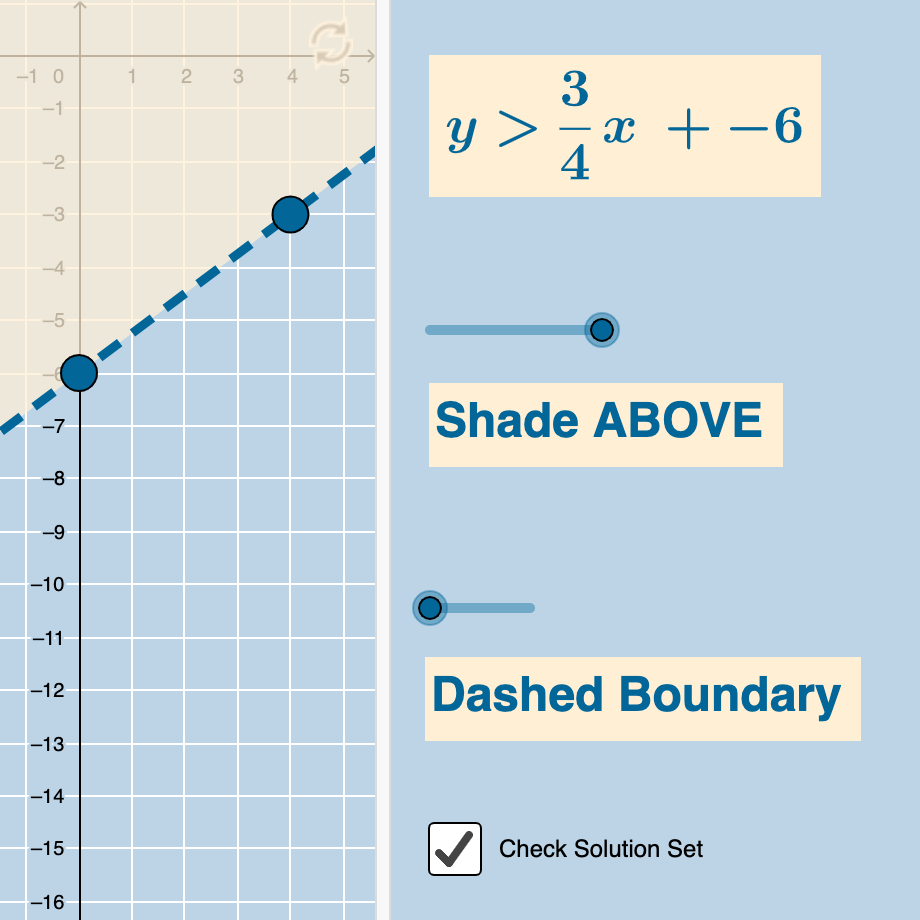Graphing Linear Inequalities with 2 Variables (Quiz)

Activity

Tim Brzezinski

•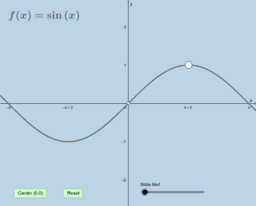Sine Identity

Activity

Tim Brzezinski

•Solving Linear Systems by Graphing: Quiz (V2)

Activity

Tim Brzezinski

•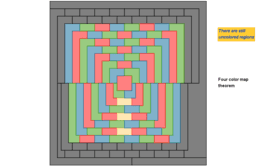Four colors theorem

Activity

•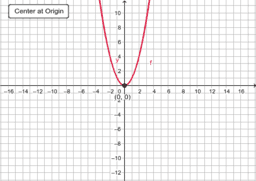Function Transformations

Activity

Mark Beckwith

•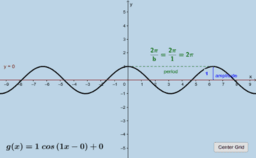Graphing Sine & Cosine Functions (II)

Activity

Tim Brzezinski

•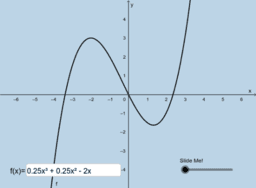Function Or Not?

Activity

Tim Brzezinski

•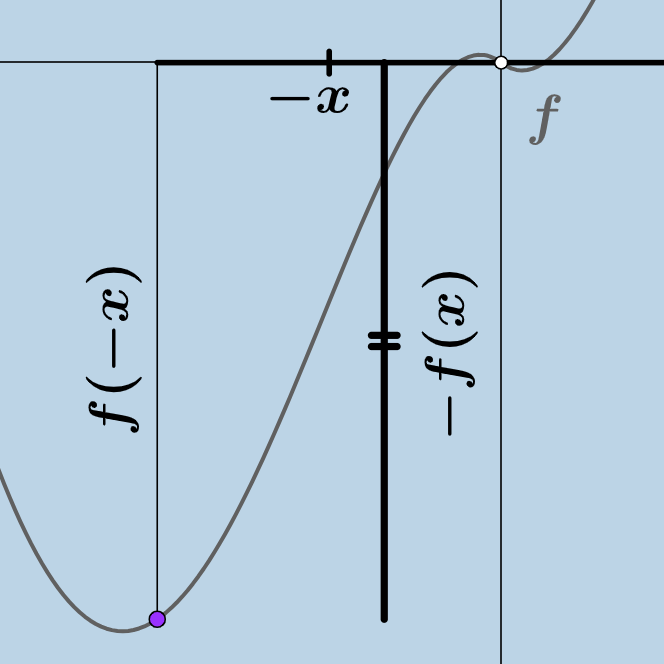Odd Functions!

Activity

Tim Brzezinski

•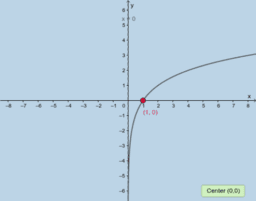Logarithmic Functions: Graphs

Activity

Tim Brzezinski

•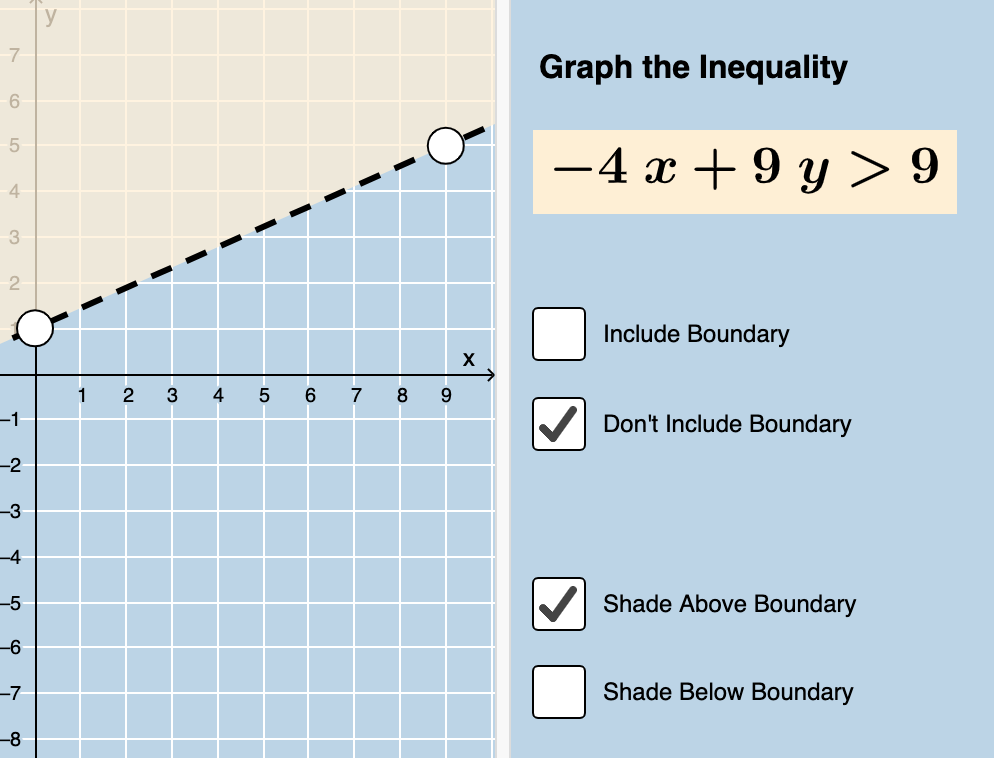Graphing Linear Inequalities Written in Standard Form (Quiz)

Activity

Tim Brzezinski

•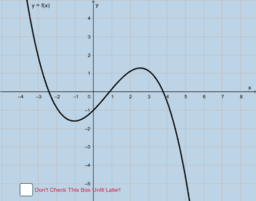Sketch the Derivative (Warm Up)

Activity

Tim Brzezinski

•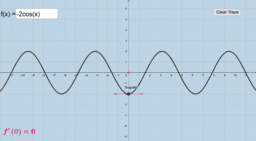Graphing The Derivative of a Function

Activity

Tim Brzezinski

•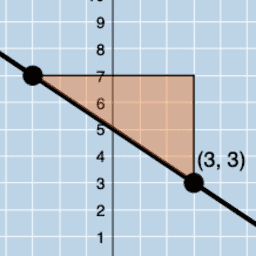Writing the Equation of a Line Given a Slope & Point (V1)

Activity

Tim Brzezinski

•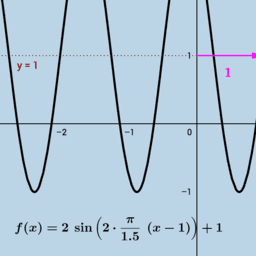Graphing Sine & Cosine Functions (I)

Activity

Tim Brzezinski

•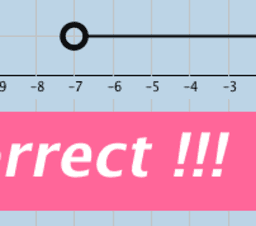Interval Notation: Quiz (Single Sets & Conjunctions)

Activity

Tim Brzezinski

•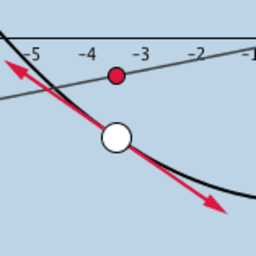Derivatives (Intro)-2

Activity

Tim Brzezinski

•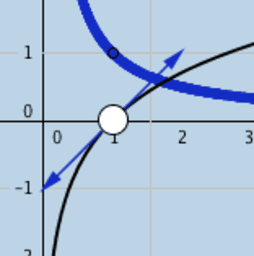Derivative of Natural Log Function

Activity

Tim Brzezinski

•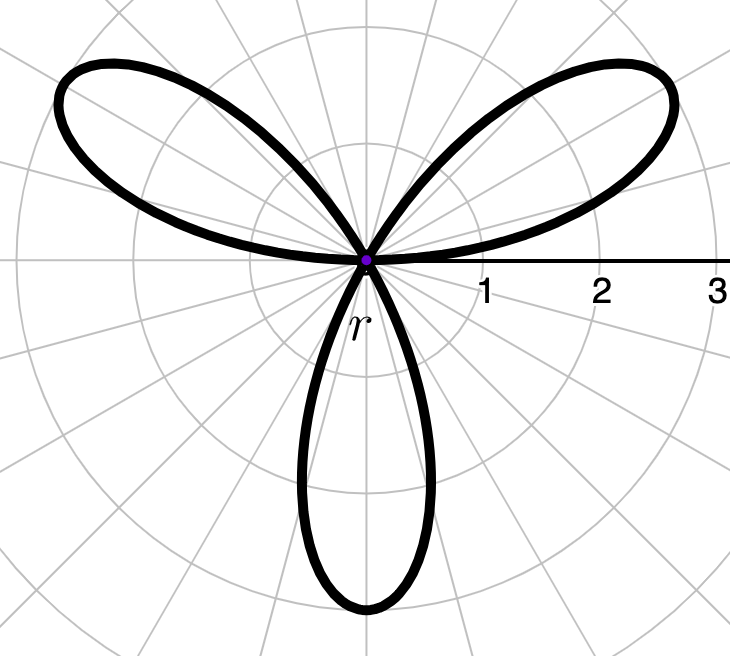Polar Function Graphing

Activity

Tim Brzezinski